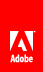# WBP-150

`XPath expression: You might be using the true() XPath function to test for node existence. To test for node existence, compare true() with the results of using the boolean()function on the node.`

This is an XPath validation message.

Explanation

Resolution

The node that an XPath expression evaluates to is being set or compared to the results of the true function. The true function returns a value of true.

To test for the existence of a node, use the boolean function. The following route condition tests whether the node for the stringVar variable exists:

`boolean(/process_data/@stringVar) = true()`

Ensure that you are using the true function correctly. (See true .)

To test the value of a boolean value, convert the value to a string. The following example converts the boolean variable named boolVar to a string, and compares it to ’true’:

string(/process_data/@boolVar) = "true"// Ethnio survey code removed